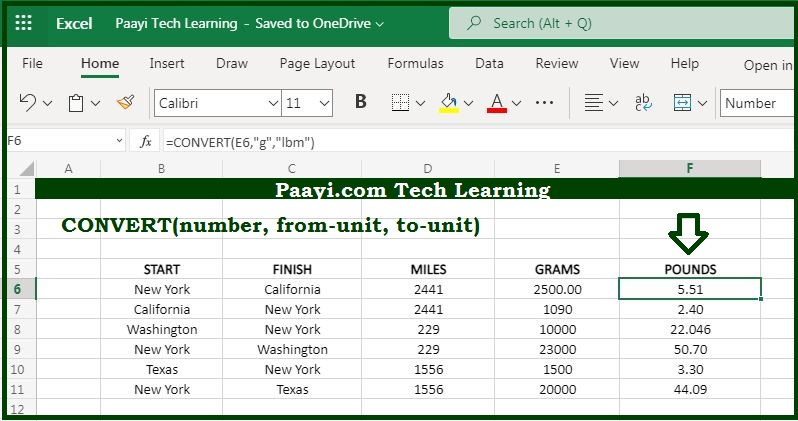# Learn How to Use Microsoft Excel CONVERT Function

Written by | 0 Comments | 659 Views

In this article, you will learn how to use the Microsoft Excel CONVERT function and its prime function in Microsoft Excel. You will also get to know the Microsoft Excel CONVERT function return value and syntax with the help of some examples.

Microsoft Excel CONVERT Function

The prime function of the Microsoft Excel CONVERT function is to covert measure units. That implies with the help of the CONVERT function you can able to convert the number from one measurement system to another. In simple words, you can able to convert feet into meters, pounds into kilograms, gallons into liters, and likewise with every other measurement unit.

Return Value of CONVERT Function

The return value will be the number in the new measurement system.

Syntax of CONVERT Function

=CONVERT(number, from-unit, to-unit)

Where the argument:

number: It is the numeric value you want to convert.

from-unit: It is the starting unit of number.

to-unit: It is the resulting unit of the number.

## How to Use Microsoft Excel CONVERT Function?As we know that CONVERT function used to convert the measurement units in number from one measurement system to another. As you can easily convert feet into meters, pounds into kilograms, gallons into liters, and likewise with every other measurement unit. You can also go through the "Measurement units table" available in the CONVERT function in every category.